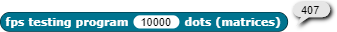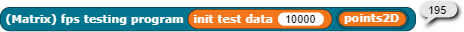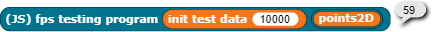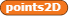# Matrix multiplication with vectors,how fast are they?

So I made a matrix multiplication project,and the central code looked like this:

I wanted to make an algorithm a little faster than this one on the measure of that fps tester.(my matrix algorithm worked 278.2ms)
But it turned out that any algorithm that was not a matrix,did not work at all.

300ms

So why could the matrix algorithm be actually faster?(which actually did a lot more extra steps,even doing that 0 on the bottom right of the rotational matrix)
btw thanks @cornelios207 making a matrix multiplication hyperblock i couldn't even optimize

what the optimization did

(the rotate then project matrix works like this)
c1c2 -s1
s1c2 c1
s2 0
(the two "optimize" algorithms just tally up the matrix times vector:)
x=c2(c1x+s1y)+s2z
y=c1y-s1x
(you could do the calculations yourself,it definitely works)

I'm confused. Why are you using trig functions to multiply matrices? That's probably where your extra time is going.

For 10k points....

MapJSBTW:

• for JS pitch/yaw must be radians
•vector of the 2D points after transformation
• code embedded

I'm trying to apply rotational matrices to 10000 points

yeah ik it was just a test so i did not care about that

Wait did you use one big HOF to process the data?Oh now it makes sense.Seems like snap laggs when going thru functions
(at first glance your thing does not work,but actually if we return the returned list it would work,because we want to do 3d engine)

Most of the time wasted in my code is the list and array conversion

This topic was automatically closed 30 days after the last reply. New replies are no longer allowed.